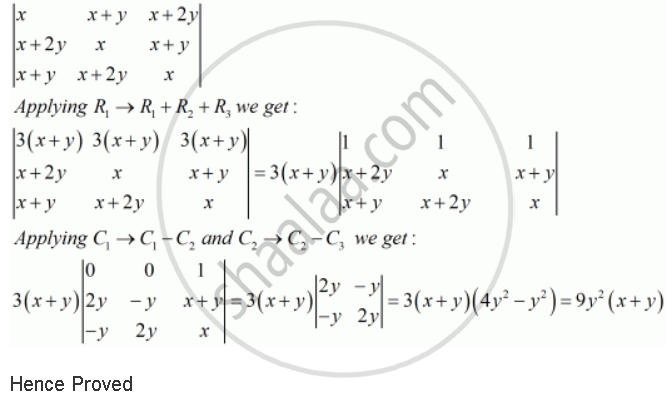Share

# Using Properties of Determinants, Prove that Matrix (X,X+Y,X+2y),(X+2y, X,X+Y),(X+Y, X+2y, X) = 9ysquare2(X + Y) - CBSE (Science) Class 12 - Mathematics

#### Question

Using properties of determinants, prove that |(x,x+y,x+2y),(x+2y, x,x+y),(x+y, x+2y, x)| = 9y^2(x + y)

#### SolutionIs there an error in this question or solution?

#### APPEARS IN

Solution Using Properties of Determinants, Prove that Matrix (X,X+Y,X+2y),(X+2y, X,X+Y),(X+Y, X+2y, X) = 9ysquare2(X + Y) Concept: Properties of Determinants.
S